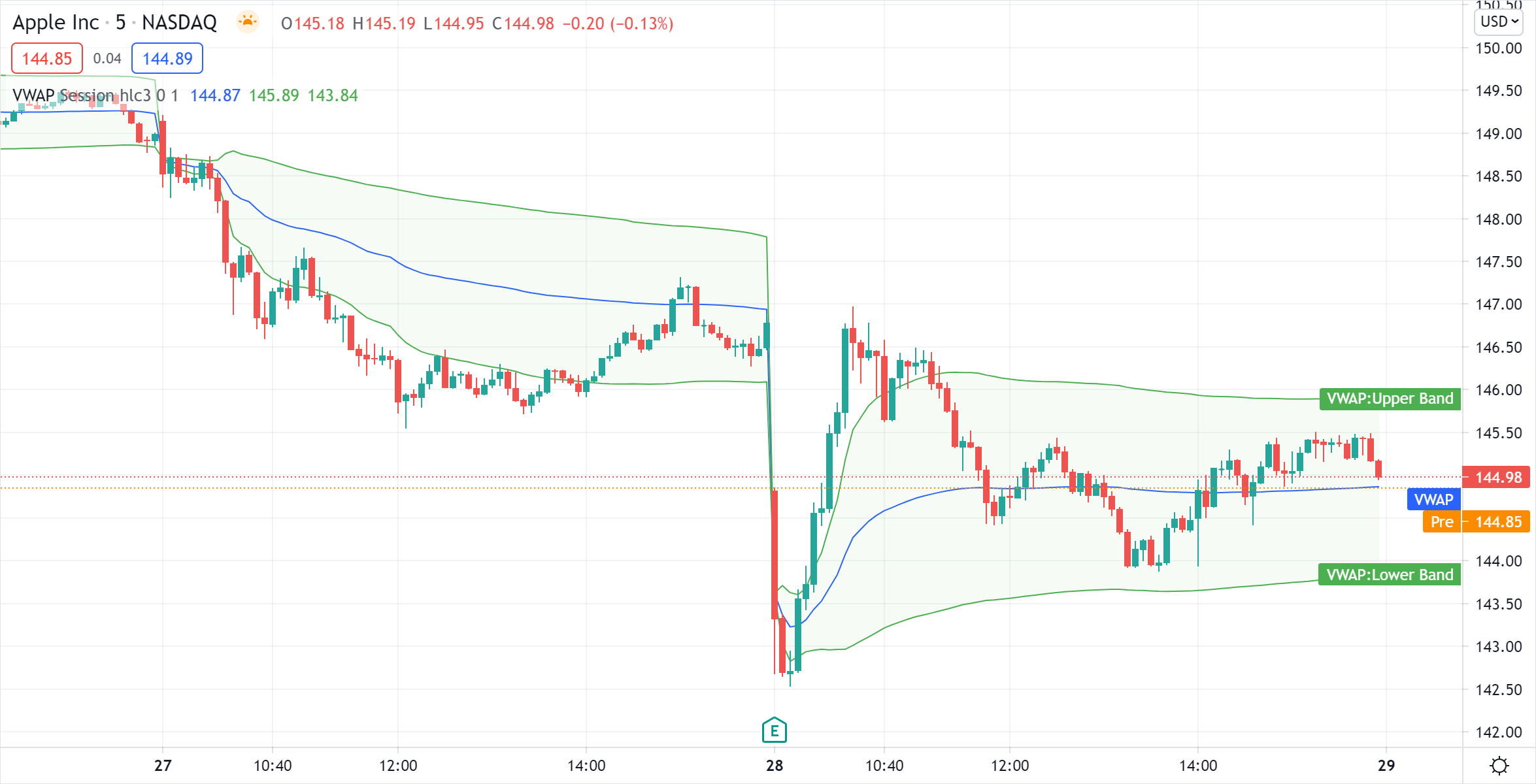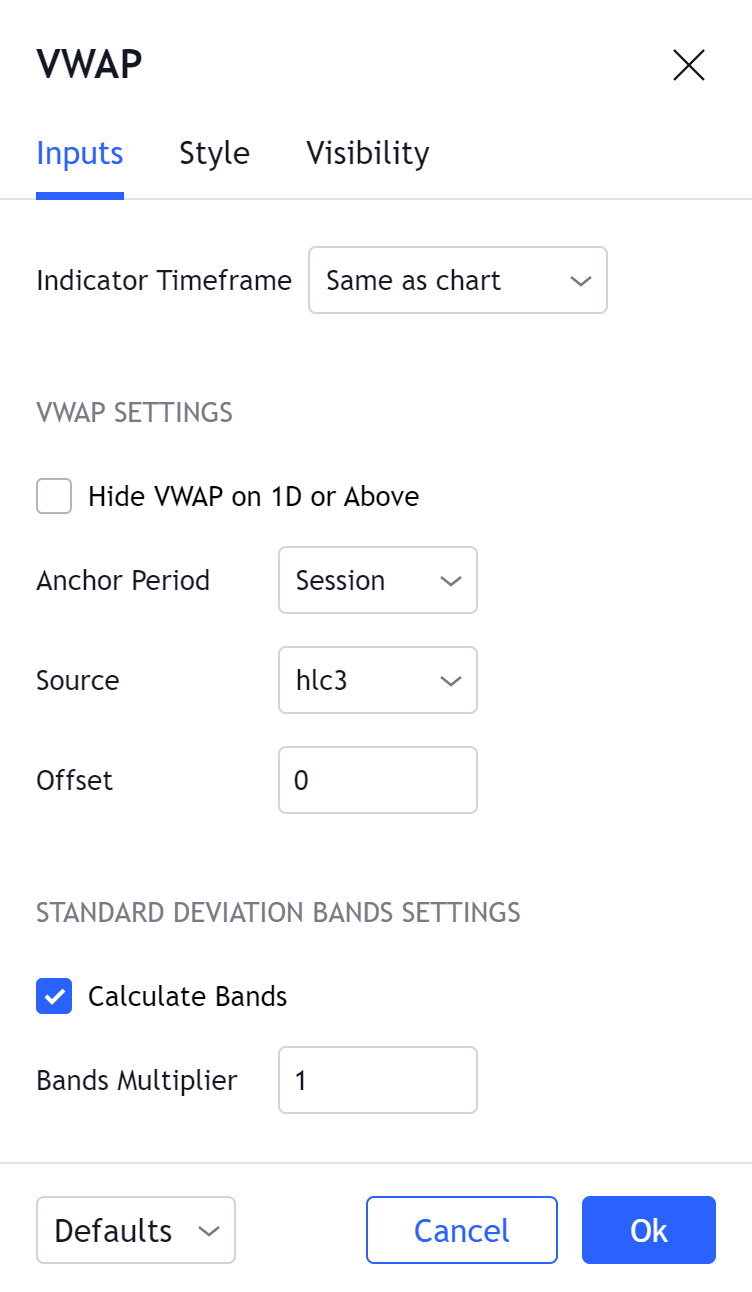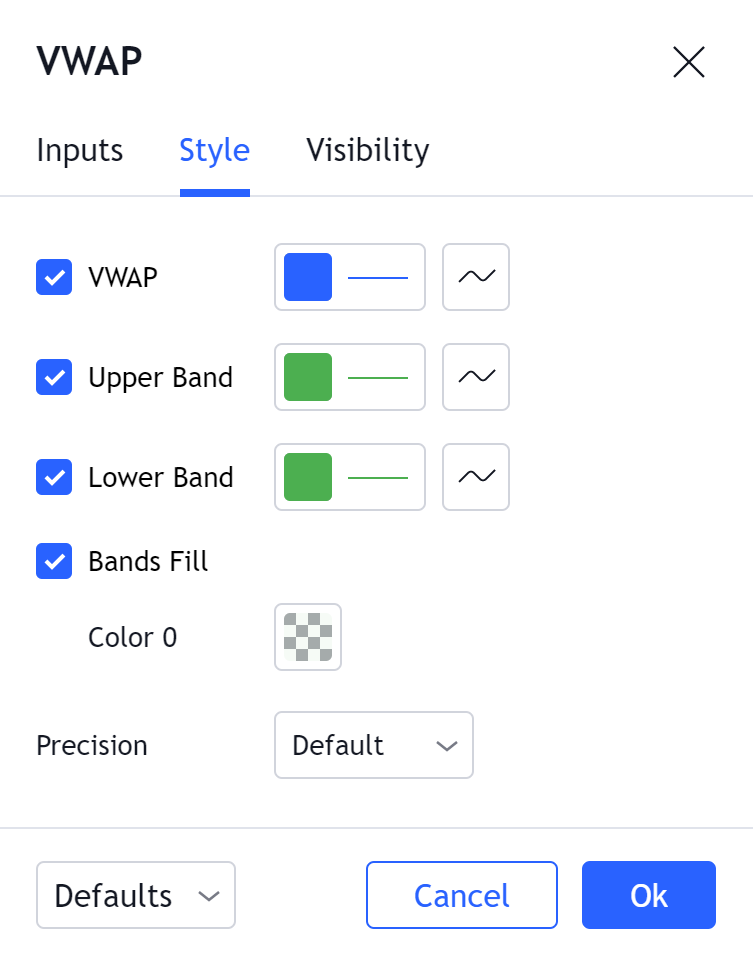# Volume Weighted Average Price (VWAP)

Volume Weighted Average Price (VWAP) is a technical analysis tool used to measure the average price weighted by volume. VWAP is typically used with intraday charts as a way to determine the general direction of intraday prices. It's similar to a moving average in that when price is above VWAP, prices are rising and when price is below VWAP, prices are falling. VWAP is primarily used by technical analysts to identify market trend.#### Calculation

There are five steps in calculating VWAP:

1. Calculate the Typical Price for the period.
`[(High + Low + Close)/3)]`
2. Multiply the Typical Price by the period Volume.
`(Typical Price x Volume)`
3. Create a Cumulative Total of Typical Price.
`Cumulative(Typical Price x Volume)`
4. Create a Cumulative Total of Volume.
`Cumulative(Volume)`
5. Divide the Cumulative Totals.
`VWAP = Cumulative(Typical Price x Volume) / Cumulative(Volume)`

#### The basics

The Volume Weighted Average Price indicator is similar to a moving average in that when prices are advancing, they are above the indicator line and when they are declining, they are below the indicator line. Keep in mind, however, that much like a moving average, VWAP can also experience lag. Lag is inherent in the indicator because it's a calculation of an average using past data.

VWAP can be used over any time frame: intraday (seconds, minutes, hours), week, month, year, decade, century. For example, if you select a weekly interval, the sum of the values will accumulate starting from the first trading day of each week.

#### What to look for

##### Trend Identification

Trend Identification is a major benefit of using the Volume Weighted Average Price indicator. The premise is very straightforward but can be very useful, especially when used for confirming trading signals.

Bullish Trend is characterized by prices trading above the VWAP.

Bearish Trend is characterized by prices trading below the VWAP.

Sideways Market is characterized by prices trading above and below the VWAP.

#### Summary

The Volume Weighted Average Price is an interesting indicator because unlike many other technical analysis tools, it's best suited for intraday analysis. It's a solid way of identifying the underlying trend of an intraday period. When price is above the VWAP, the trend is up and when it's below the VWAP, the trend is down. There is a downside, however. Even though it is primarily used on an intraday basis, there can still be a great deal of lag between the indicator and price. The indicator begins calculating at the open and stops calculating at the close. Therefore, for a chart using a short timeframe (i.e. 1 minute), there can be several hundred periods within that single day. The closer it is to the day's close, the more lag the indicator will have. This is true for any indicator that calculates an average using past data.

Inputs##### Indicator Timeframe

Specifies the timeframe that the indicator is calculated on. This option allows calculating VWAP based on a data from another timeframe, e.g. having VWAP calculated on 1H chart be displayed on a 5m chart.

##### Hide VWAP on 1D or Above

If selected, VWAP will only be displayed on intraday timeframes. This is useful with the 'Session' Anchor Period, because VWAP makes sense only when the Anchor Period is higher than the chart timeframe.

##### Anchor Period

Indicator calculation period. This setting specifies the Anchor, i.e. how frequently the VWAP calculation will be reset. For VWAP to work properly, each VWAP period should include several bars inside of it, so e.g. setting Anchor to 'Session' and timeframe to '1D' is not useful because the indicator will be reset on every bar.

Possible values: Session, Week, Month, Quarter, Year, Decade, Century, Earnings (reset on earnings), Dividends (reset on dividends), Splits (reset on splits).

##### Source

The source for the VWAP calculation. Traditionally, bar's average value is used as the source. By default, the source is hlc3, but hl2 is another common option.

##### Offset

Changing this number will move the VWAP either Forwards or Backwards, relative to the current market. Zero is the default.

##### Calculate Bands

If selected, the indicator will calculate the Standard Deviation of the all VWAP values since the last anchor.

##### Bands Multiplier

The value the Standard Deviation bands will be multiplied by before being plotted on the chart.

#### Style##### VWAP

Can toggle the visibility of the VWAP as well as the visibility of a price line showing the actual current value of the VWAP. Can also select the VWAP Line's color, line thickness, and line style.

##### Upper Band, Lower Band

Can toggle the visibility of the VWAP standard deviation bands and set their colors and line types.

##### Bands Fill

Can change whether to fill the space between the standard deviation bands and tune the color

##### Precision

Sets the number of decimal places to be left on the indicator's value before rounding up. The higher this number, the more decimal points will be on the indicator's value.